Video Transcript of:

Navigating Intrinsic Triangulations - SIGGRAPH 2019

(Authors: Nicholas Sharp, Yousuf Soliman and Keenan Crane)

Transcript by http://rodolphe-vaillant.fr

Related:

SIGGRAPH 2021 COURSE – “Geometry Processing with Intrinsic Triangulations”

Course pdf

Course video

Course code tutorial

SOURCE CODE

https://github.com/nmwsharp/navigating-intrinsic-triangulations-demo

https://geometry-central.net/

This is a manifold mesh and it faithfully reproduce the intended shape:However, because of the thin and long triangles or inconsistent vertex sampling many geometry processing algorithms would fail / not converge.

Re-sampling is not ideal: you may introduce too many faces/vertices (slow to process) or degrade the original shape: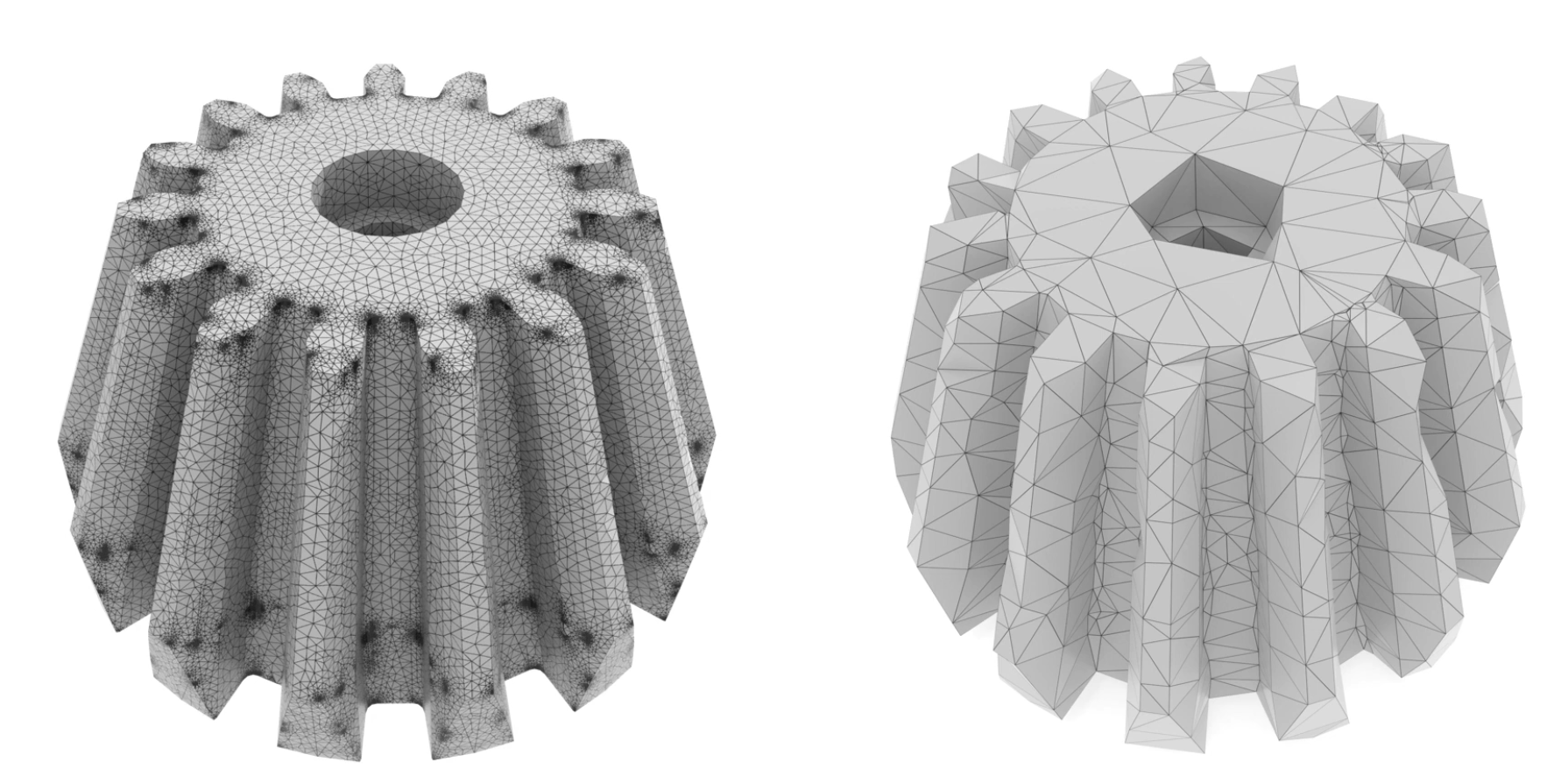The problem is we are coupling 2 different problems: faithfully representing the shape of the object and processing that shape with the same data structure. So we have the same triangulation for both processing and shape representation.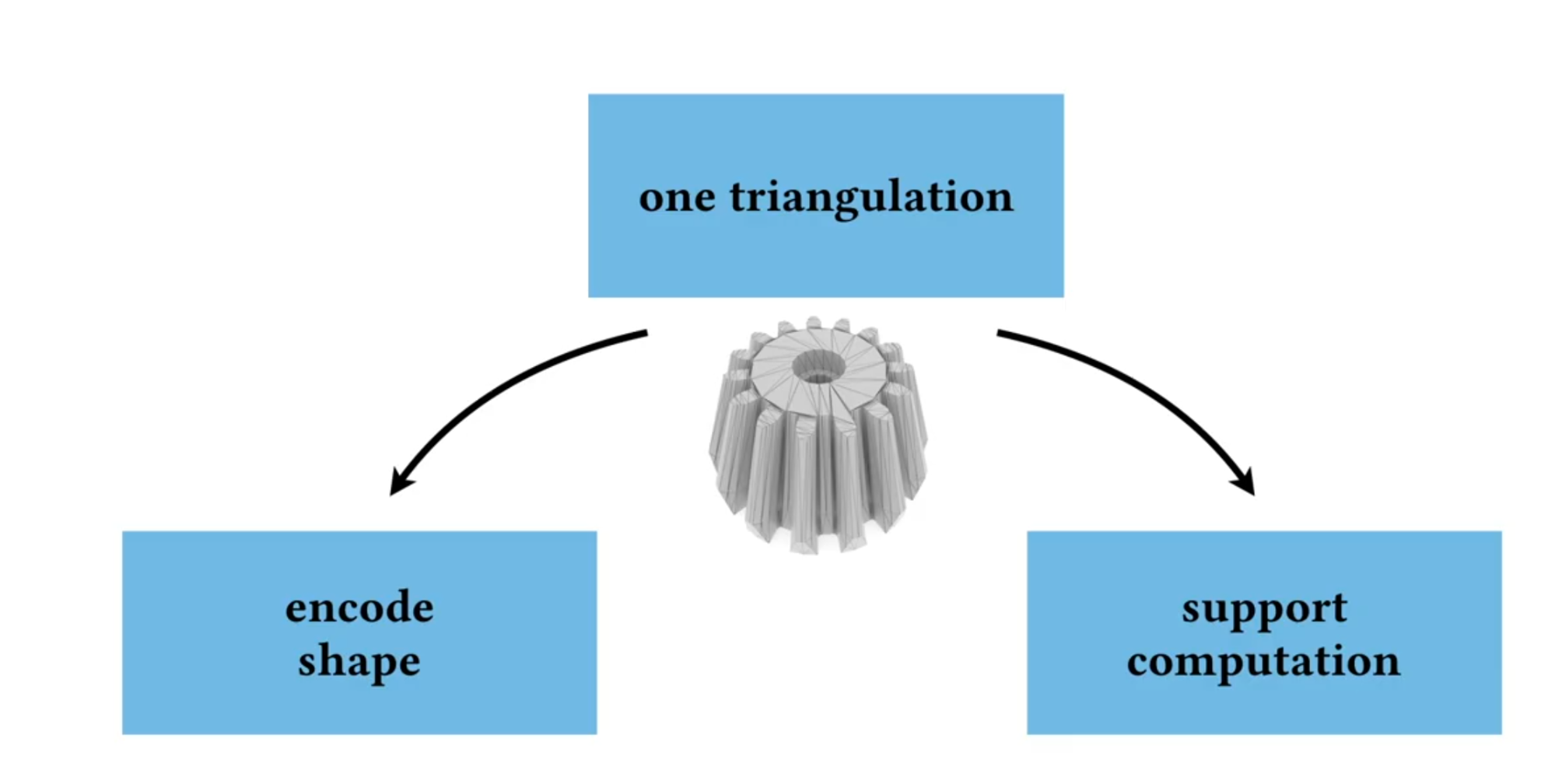They argue that separate triangulations should be used for each task:  “Shape encoding” and “processing”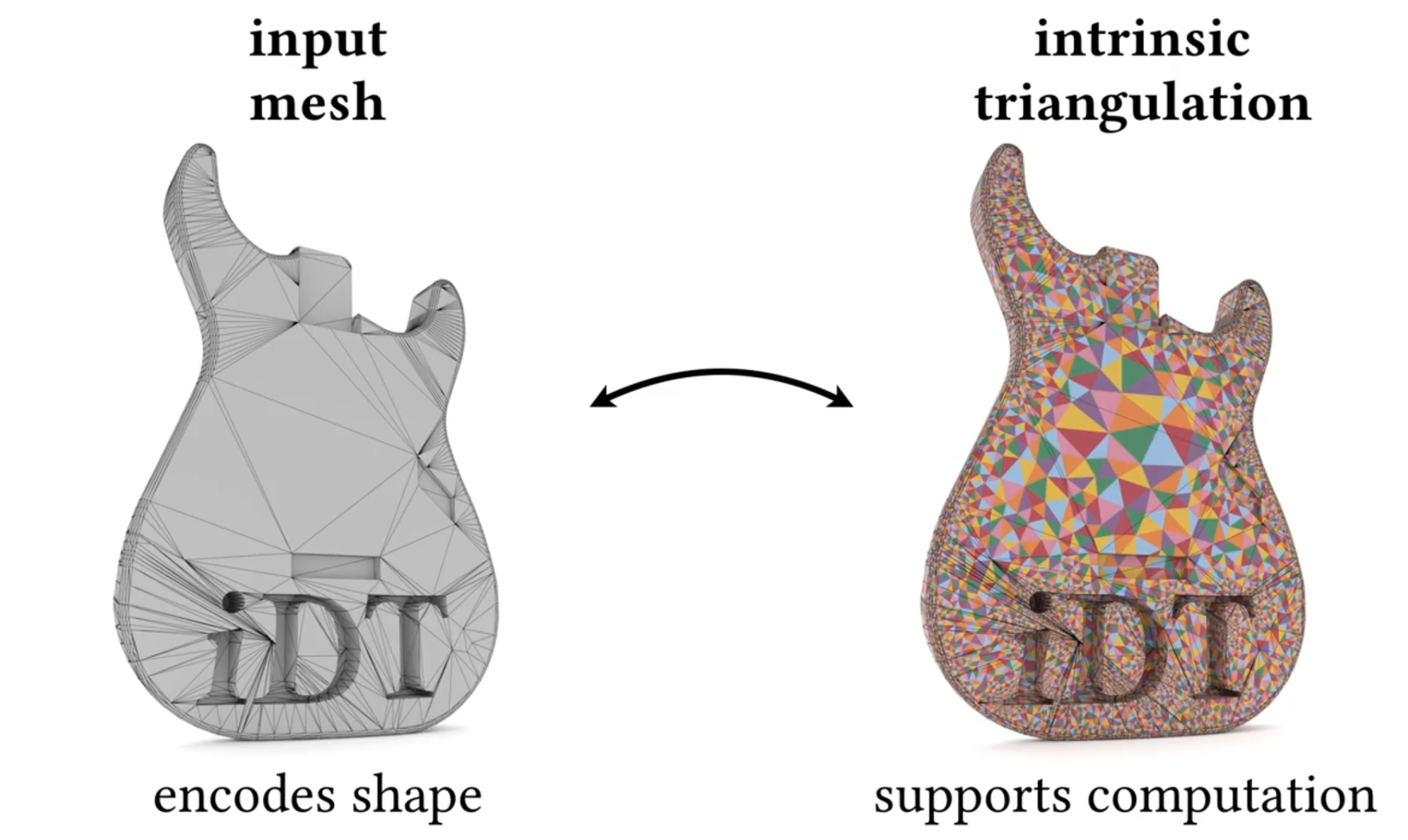Just by having a nice triangulation catered towards geometry processing you can easily reuse past algorithms and make them more robust while still retaining the original geometry 😊 Moreover the construction of this new triangulation (intrinsic triangulation) is fast.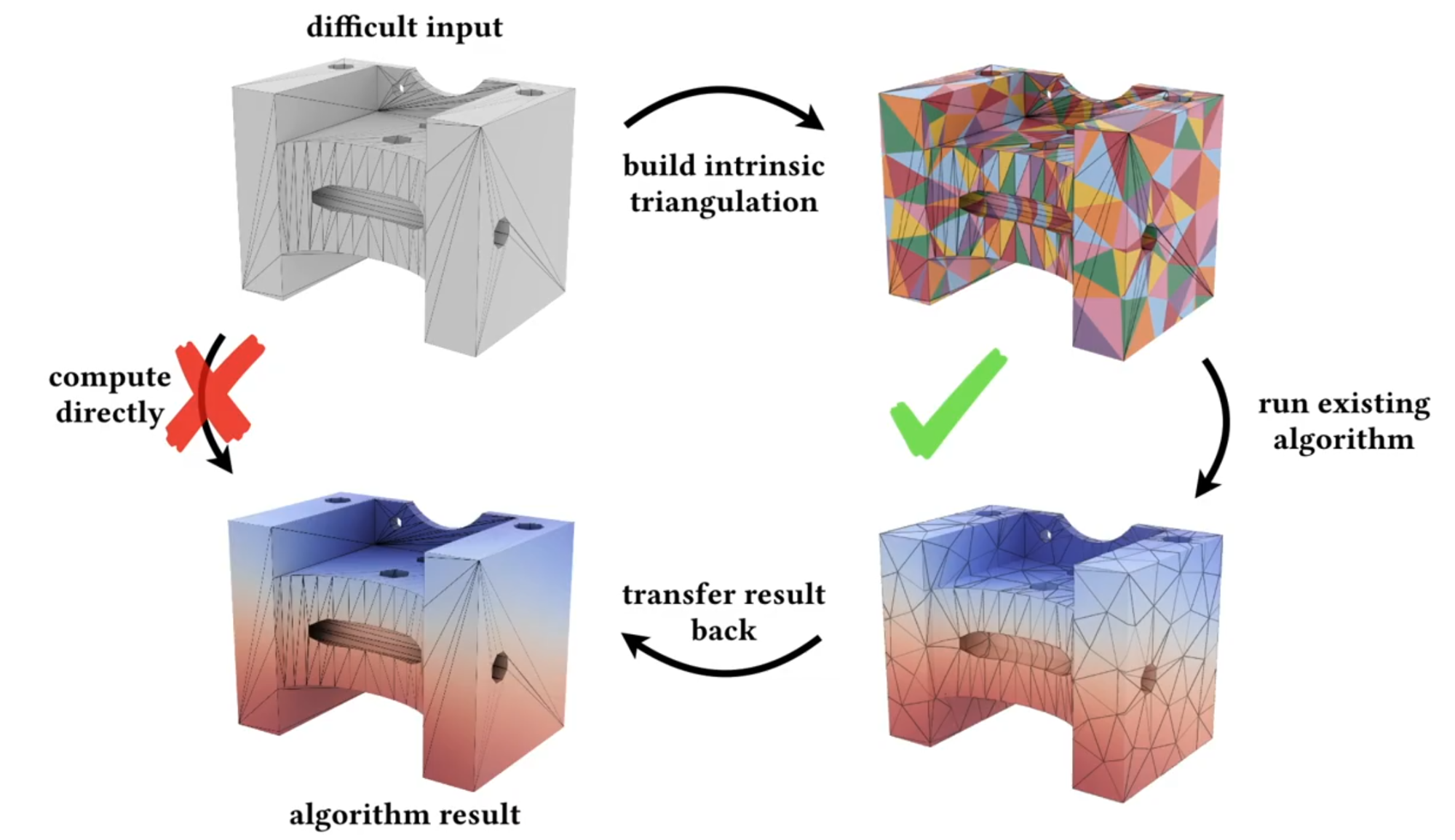So just what are these intrinsic triangulations? Well let's remember what makes a triangle. A Euclidean triangle is one that has straight edges and a flat interior, and as we all know, once you know the three edge lengths of a triangle, its shape is defined. Other quantities such as its area, its angle, its cotangent weights and so forth can easily be computed directly from these edge lengths.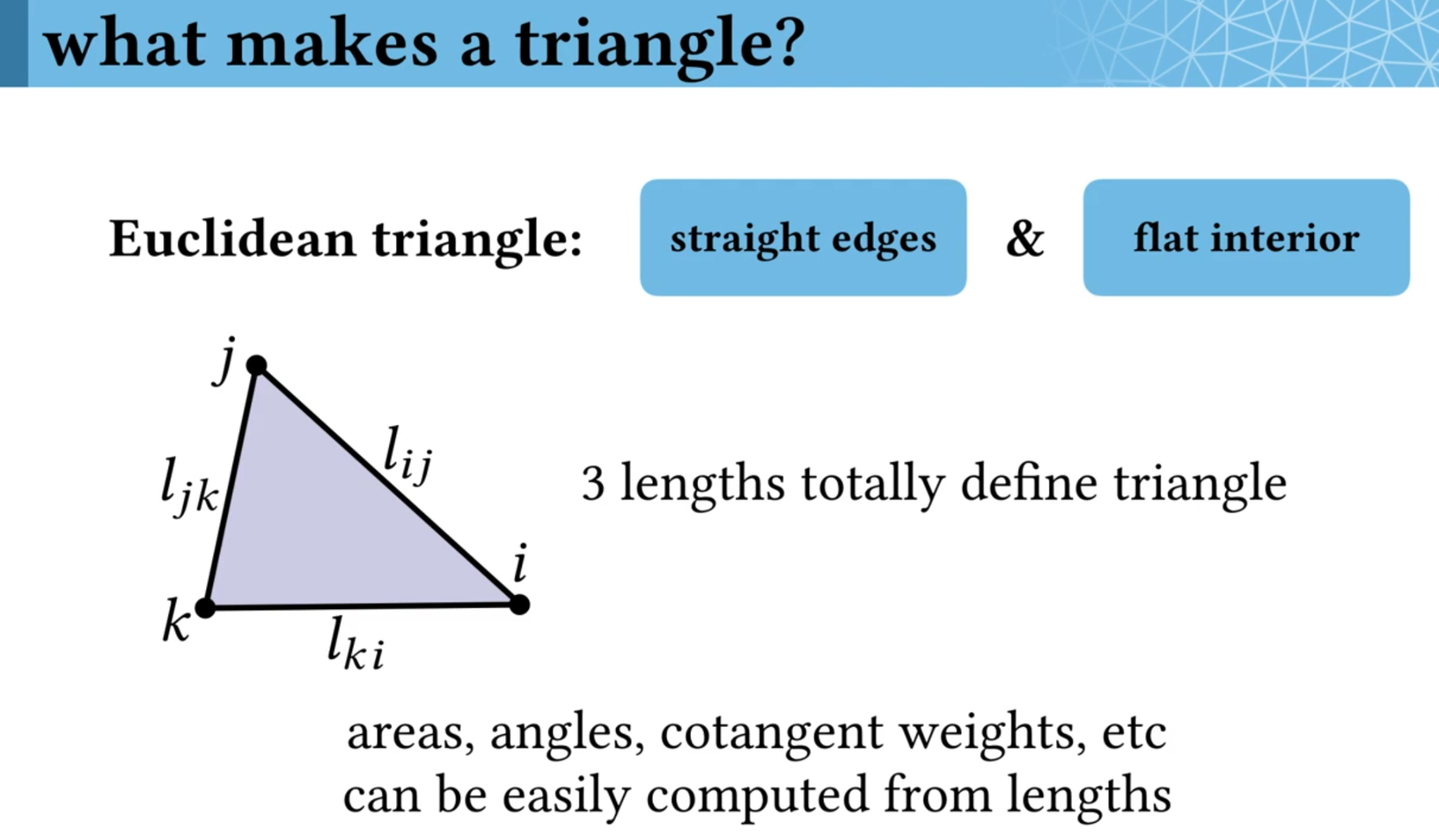I'm gonna argue that this triangle here meets our definition: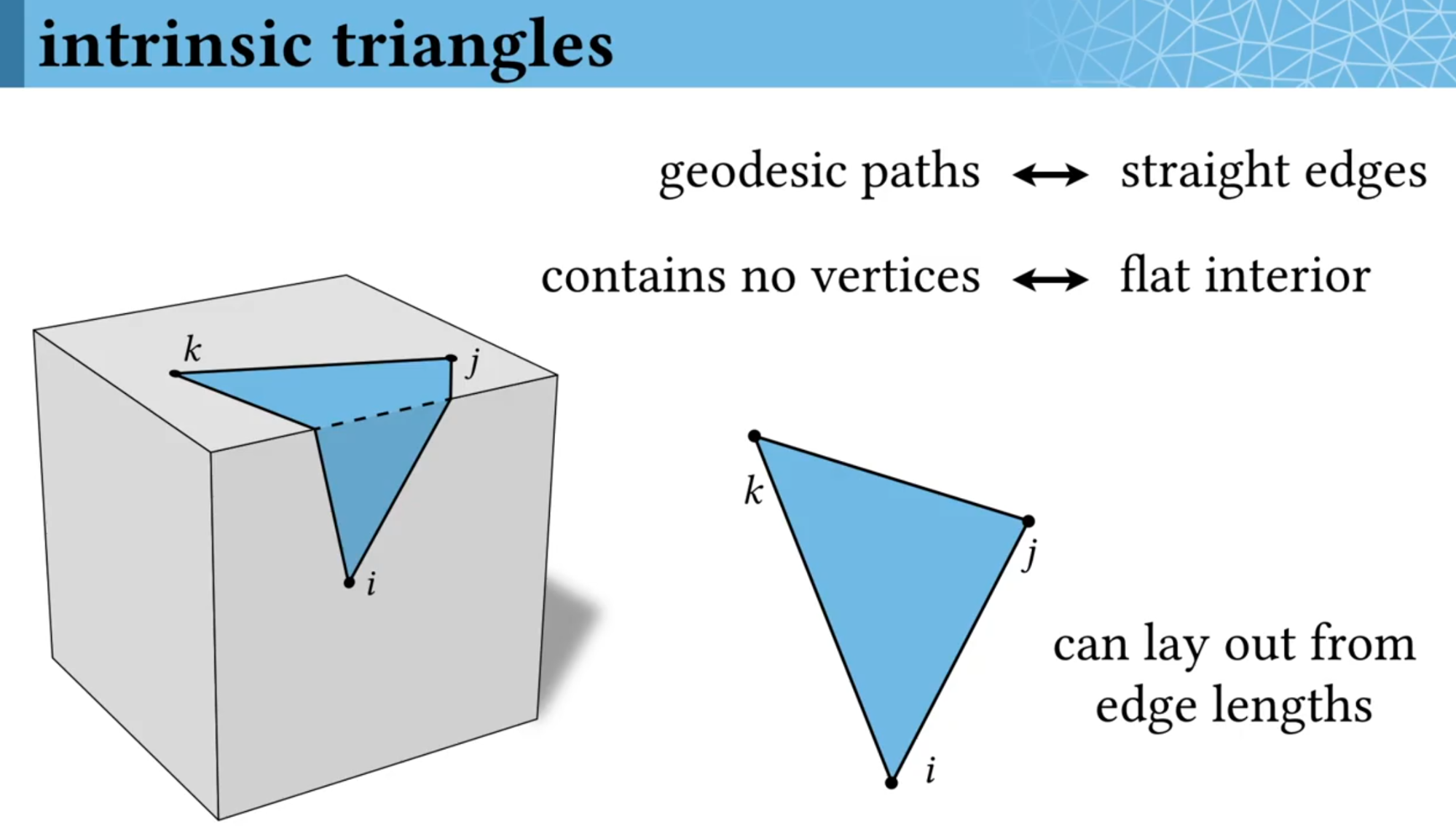It may look bent but its edges are geodesic paths across the surface.

• Which means they're straight
• There are no vertices on the interior of the triangle which means it as a flat interior

it may seem bent but we can lay it out in the plane by these three edge lengths and other quantities like areas angles and so forth can easily be computed and do not change from the bend configuration

That tells us that this bent triangle is really a perfectly good Euclidean triangle. Because everything's Euclidean you can use good old-fashioned linear elements and piecewise linear interpolation on this kind of triangle

of course you can keep going and triangulate a whole shape you're probably used to seeing triangulations of the cube like this: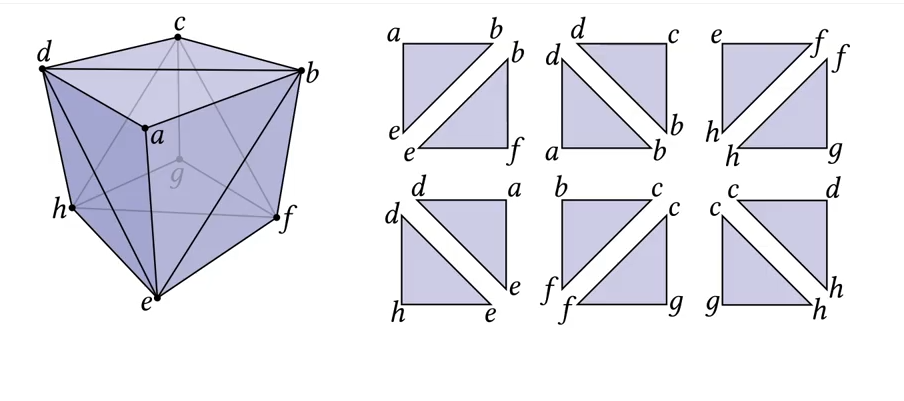but there are other more interesting intrinsic triangulations of a cube which encode the exact same shape but make use of these bent triangles: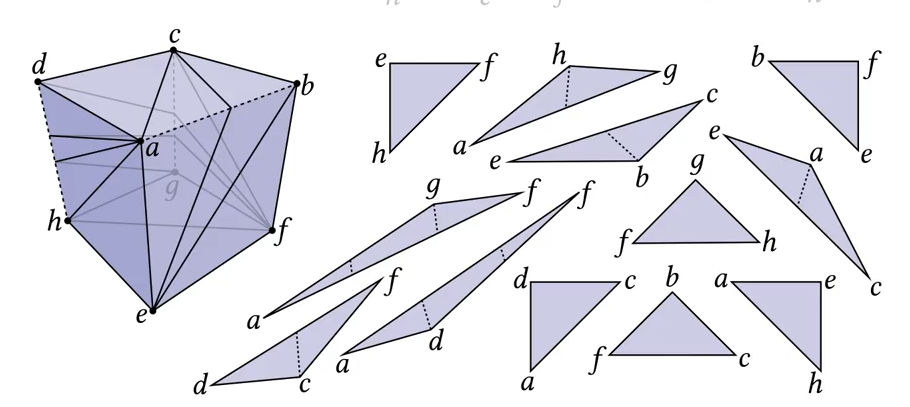So then the question is algorithmically how do you represent these interesting intrinsic triangulations. well there have really only been two previous approaches.

A first idea is to store only the mesh connectivity and edge lengths of the intrinsic triangulation: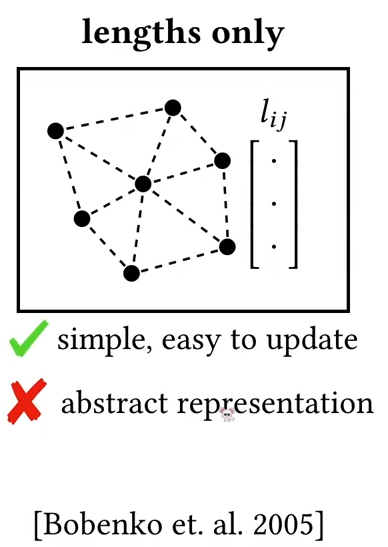This is an elegant and easy to work with but unfortunately important information has been lost you no longer know the relationship to the underlying surface.

A second idea is to explicitly store every location where the underlying mesh in the intrinsic triangulation intersect: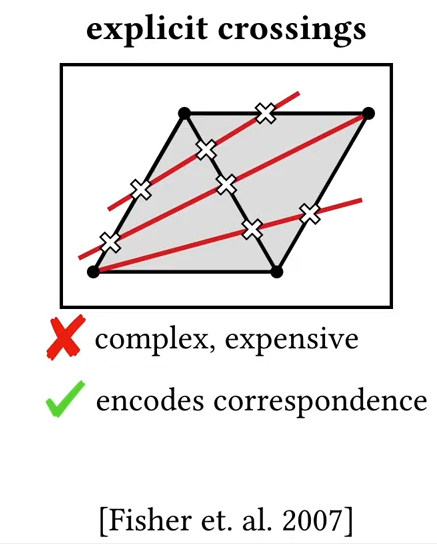This is informative but it's cumbersome and expensive to work with

We've come up with a new signpost data structure which gets the best of both worlds it's abstract and easy to wo rk with like the lengths but it's also a complete representation which will allow us to do pretty much anythingas a bonus it becomes very easy to represent problems involving tangent vectors

The core idea of our data structure is very simple we store not just the length of every intrinsic edge but we augment this with the direction of every intrinsic edge from each end point end point we call this data signposts because for each edge it tells you the direction and distance to walk in order to trace out an intrinsic edge across the surface

In particular we store these angles in tangent space with respect to a fixed arbitrary reference direction at each vertex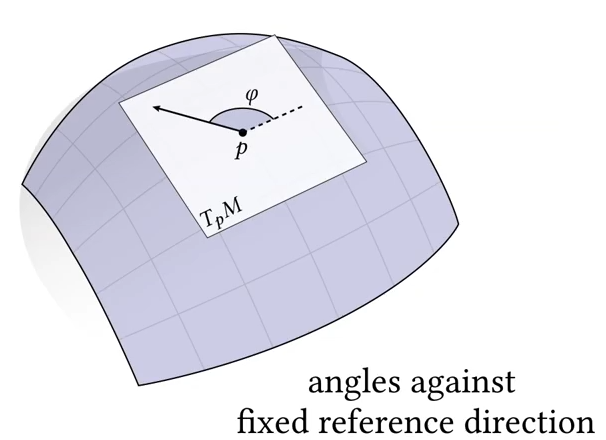To be really concrete about the data we store in our signpost intrinsic triangulations. We keep track of the connectivity of the intrinsic mesh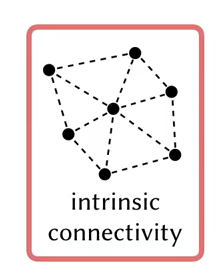the location of each intrinsic vertex (as a point on the original surface)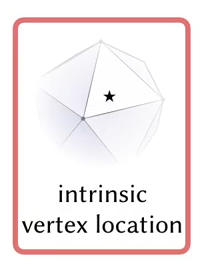the length of each intrinsic edge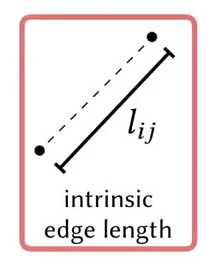and the direction of each intrinsic edge from each of its endpoints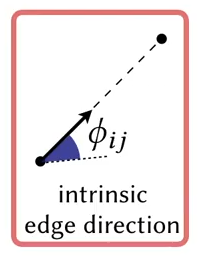In short: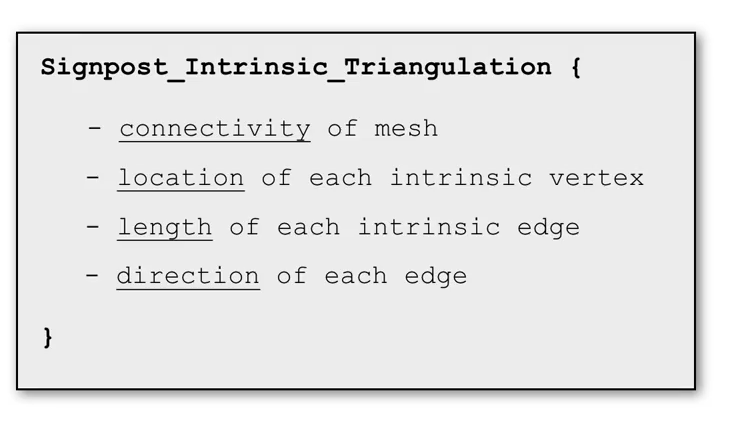This representation is implicit. Its complexity depends only on the number of intrinsic triangles, not on how the intrinsic triangulation overlaps the input mesh.

This implicit representation can be made explicit by tracing out these edges along the surface which we do lazily only when needed.This tracing is a cheap and easy operation, it just amounts to a whole bunch of 2d line line intersections

A big part of our contribution is to describe algorithms from any standard mesh operations on these intrinsic triangulations.

Across the board the implicit representation makes it easy to implement these operations and efficient too.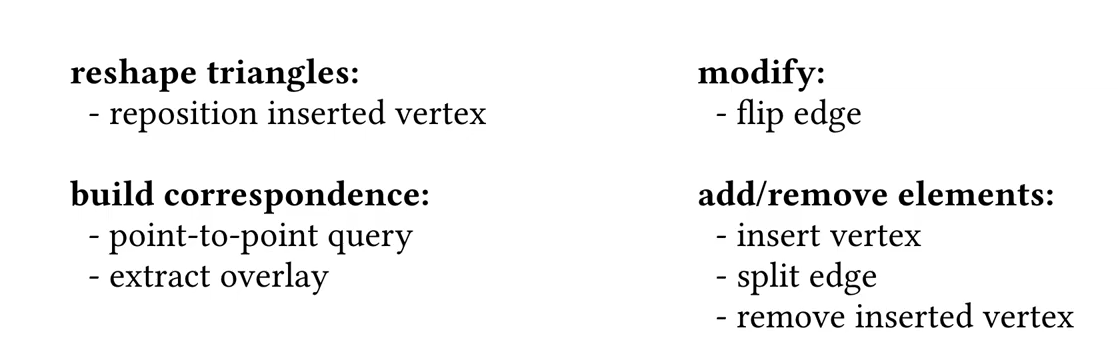Let's walk through some mesh operations on intrinsic triangulations using our signpost data structure.

The most basic operation is an intrinsic edge flip where you take one edge of an intrinsic triangulation and flip it like you see on here: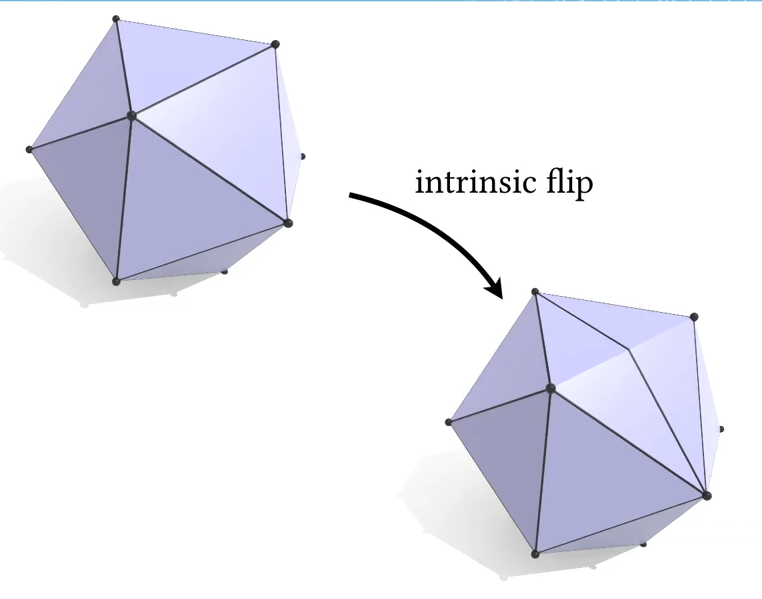to be really clear I don't mean an extrinsic edge flip which would degrade the geometry of the shape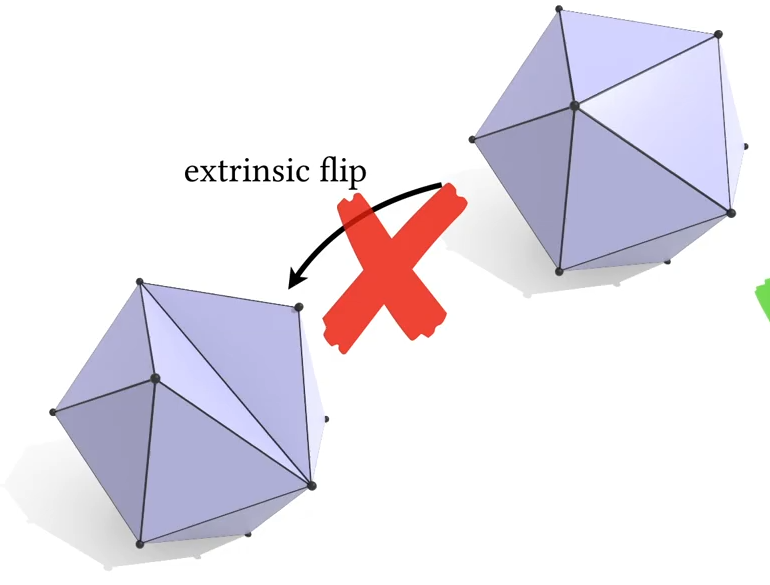I mean an intrinsic edge flip which preserves the geometry but creates a “bent” edge.

To evaluate an intrinsic edge flip in our signpost data structure will first take the edge to be flipped and lay out both of its adjacent triangles in the plane from their edge lines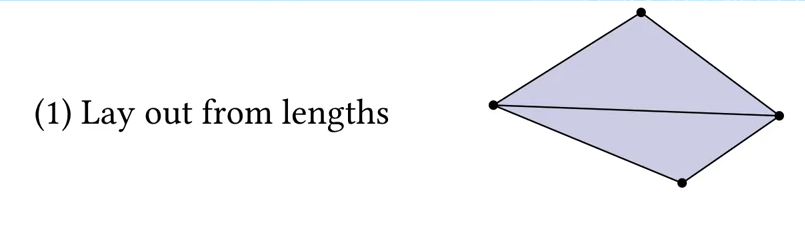we can then measure the length of the new edge which is going to be created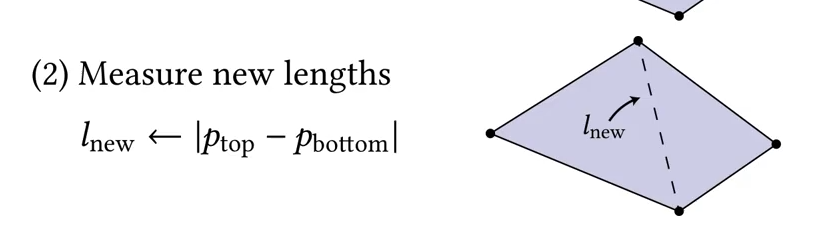and we can measure the direction of this new edge from each of its endpoints by taking an offset from some neighboring direction( which we already know)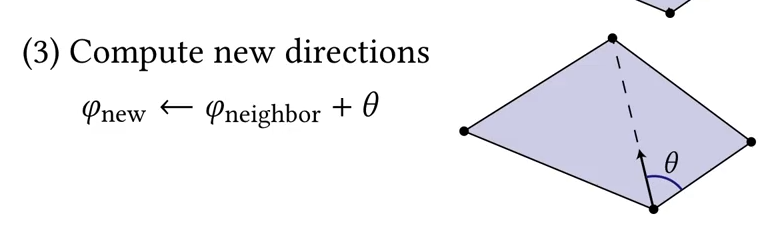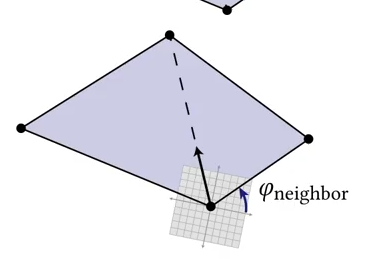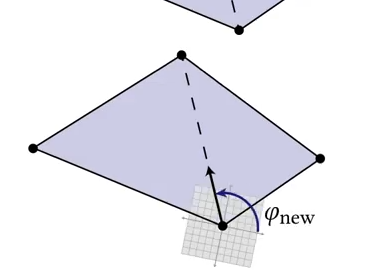finally we can update the connectivity of the intrinsic mesh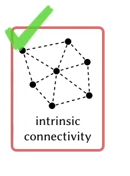The same procedure applies even if we're deep in some complicated intrinsic triangulation which has some crazy overlap with the original shape. What’s more it's always a constant time operation no matter how complicated the intrinsic triangulation is.

We can also insert new vertices into our triangulation this was prohibitively complex to do with previous data structures. Given barycentric coordinates for a new vertex in some intrinsic face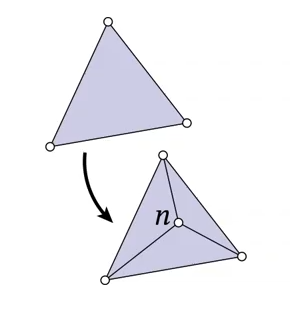we can first layout that face from its three edge lengths just as before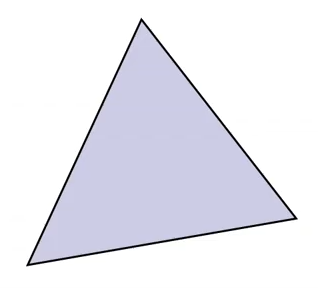and place the new vertex in this layout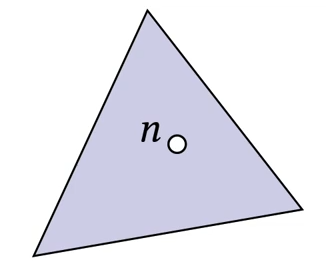we can then measure the lengths of all the new edges we need to createand compute their direction just like before by computing an offset from some known neighboring direction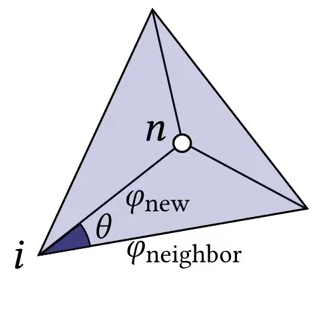one tricky detail is that we need to find the location of this new vertex on the original surface. Just knowing it's barycentric coordinates in an intrinsic triangle doesn't immediately tell us its location on the original surface. but we can get that by just tracing out one of the edges whose length and direction we just determined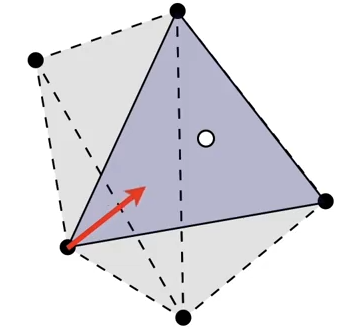wherever that tracing ends is the location of this new vertex on the original surfaceand finally we again update the connectivity of the intrinsic mesh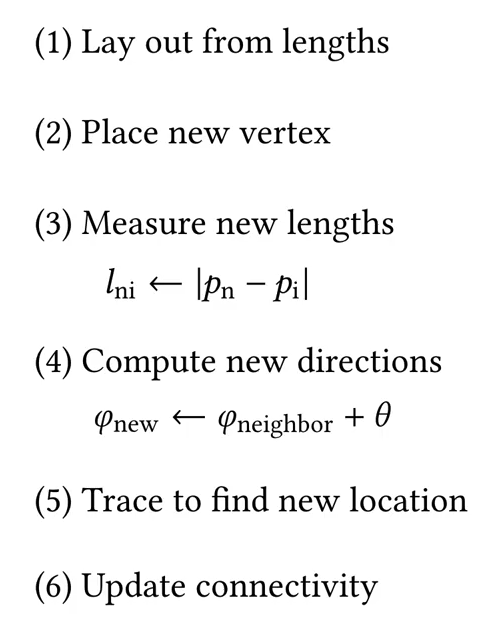notice that this procedure preserved all of the invariants of our signpost data structure, each triangle is a flat Euclidean triangle with geodesic edges and no curvature on its interior

I won't go through all of these in detail but we can do pretty much all of the standard mesh operations you would expect as well as evaluating correspondence between points on the input surface and points on the intrinsic mesh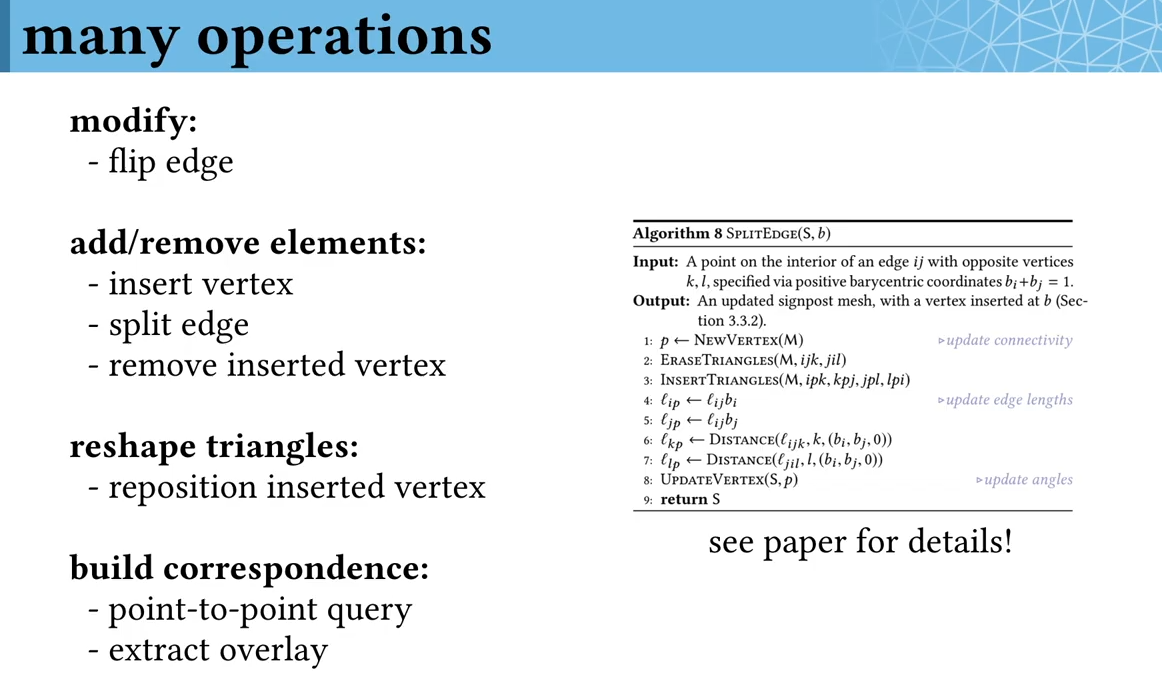one thing to notice is that one operation we never do is we never remove the original vertices of the input shape that's because those original vertices have a curvature at them and we need to maintain the invariant that all of our intrinsic triangles have no curvature on their interior.

now that we have a simple and effective data structure for representing intrinsic triangulations we can start building interesting and useful triangulations.

the consistent theme here will be that algorithms from the plane already work extremely well on surfaces under our framework.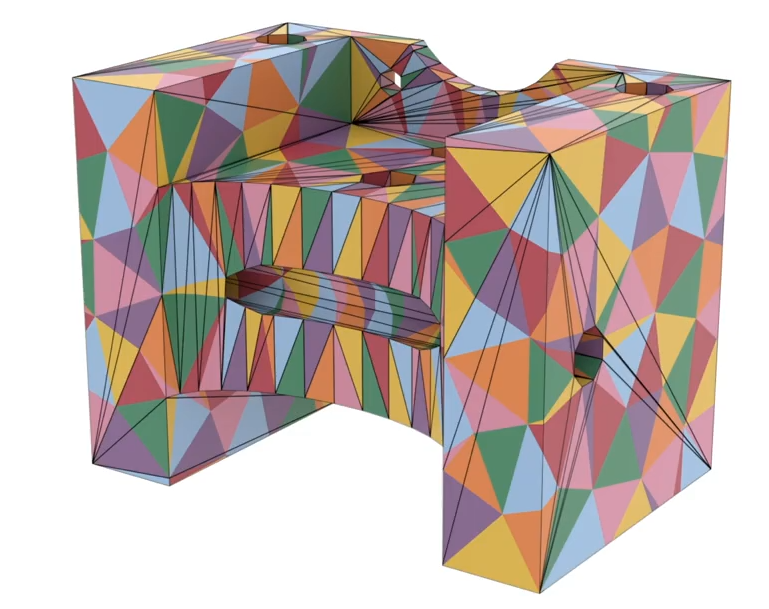Before we get too deep, a quick key about how to read our figures of intrinsic triangulations:

• The black wire frame edges denote the original input mesh
• the bright colored triangles are the faces of the intrinsic triangulation that we're working with

Of course the mesh operations we just outlined are extremely general and open the door to a wide range of possible remeshing algorithms. It just so happens that many of the best remeshing algorithms from the plane make use of the Delaunay property.

We studied three different algorithms in this framework

• intrinsic Delaunay triangulations
• intrinsic Delaunay refinement
• optimal Delauney triangulation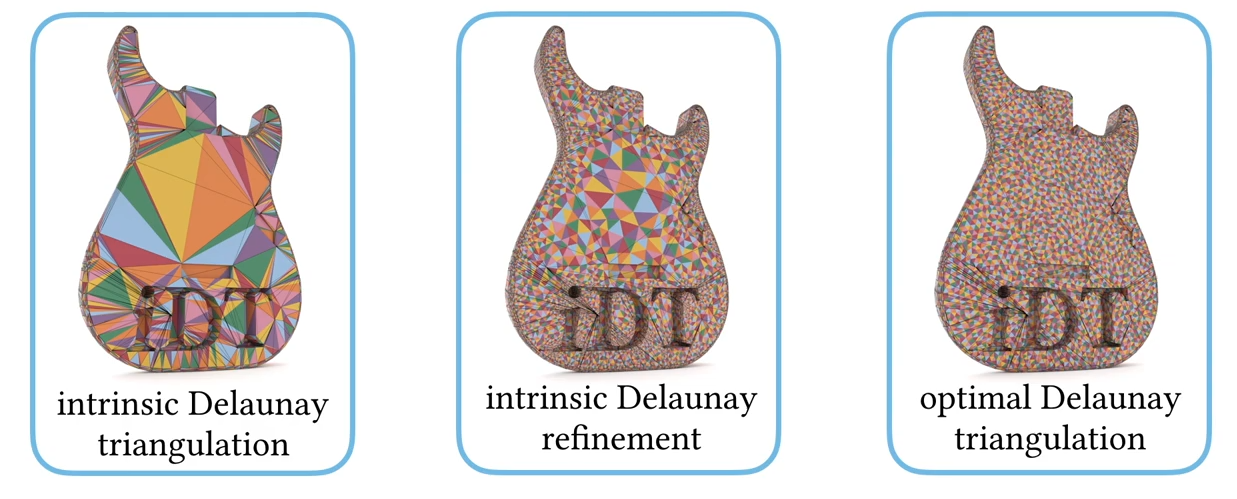in all cases will see that these methods generate meshes which have

great computational properties (high quality)

don't require adding too many elements (few elements)

and do all of this well exactly preserving the geometry Delauney triangulations (preserve exact geometry)

Good Laplacian:

Delaunay triangulations are fundamental objects in computational geometry,

among other things they guarantee that the Laplace operator has all positive edge weights and a maximum principle.

There are a lot of equivalent characterizations of Delaunay triangulations in the plane, the one that's useful for us we'll be that for every edge the opposite triangle angles sum to less than PI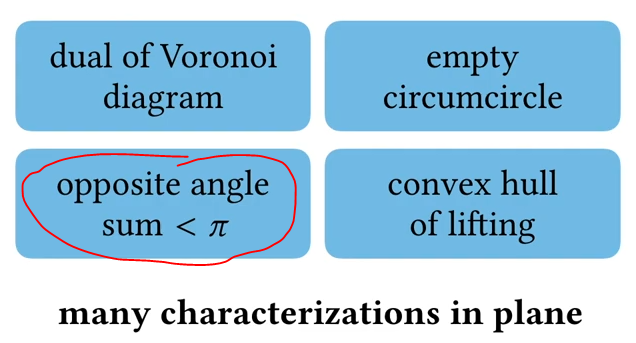on an intrinsic triangulation we can evaluate this definition by simply laying out the two triangles on either side of an edge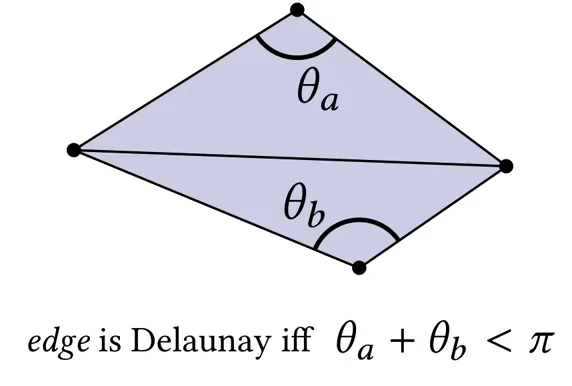and measuring those two angles in the plane we'll say that a triangulation is Delaunay triangulation if all of its edges are Delaunay.

there's a wonderfully straightforward algorithm for producing intrinsic Delaunay triangulations:

Alg:

while any edge is not Delaunay flip it

and that's it! A surprising and beautiful result is that this simple procedure will always yield the unique intrinsic Delaunay triangulation

Here's an example of running this procedure on a CAD model.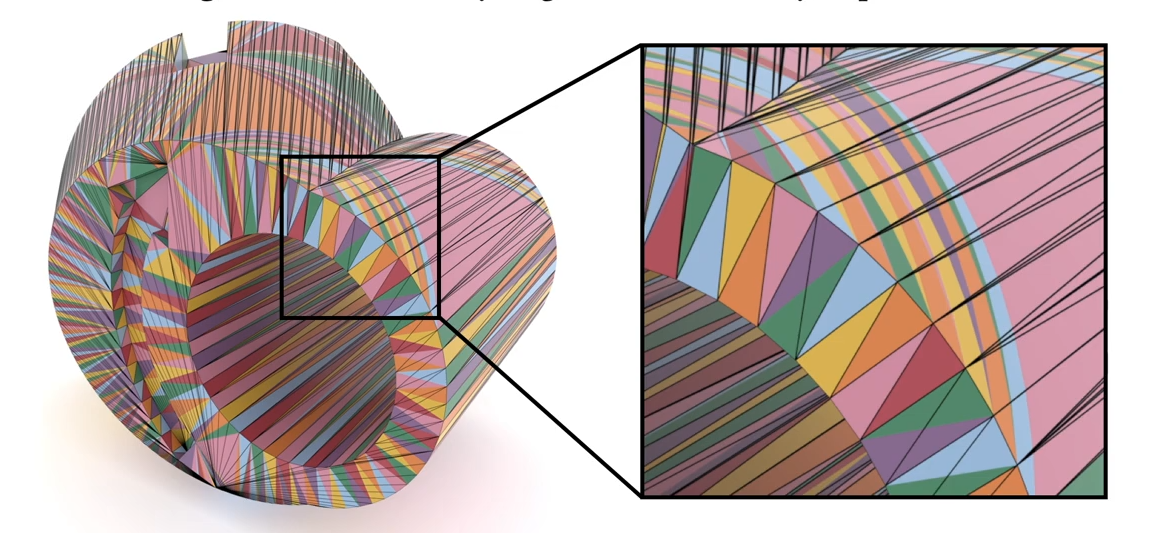Notice that the intrinsic triangulation can be very different from the mesh that you started with and might involve lots of complicated and interesting intrinsic triangles.

from the theory side the worst-case performance of this algorithm is still an open question but I can assure you that in practice it's extremely fast and effective we ran this intrinsic edge flipping procedure on each manifold mesh from the thingy 10k dataset here on this plot the x-axis is the number of edges in each mesh and the y-axis is the number of flips it took to make it intrinsic Delaunaynotice that the trend is extremely linear and there are no outliers where the number of flips required was more than a small factor of the number of edges and this is even on CAD models which are typically the hard inputs for these kinds of algorithm. generally speaking these Delaunay edge flips take less time than reading a mesh from disk

Our implicit signpost data structure is up to 30 times faster at this procedure than past explicit methods on difficult inputs.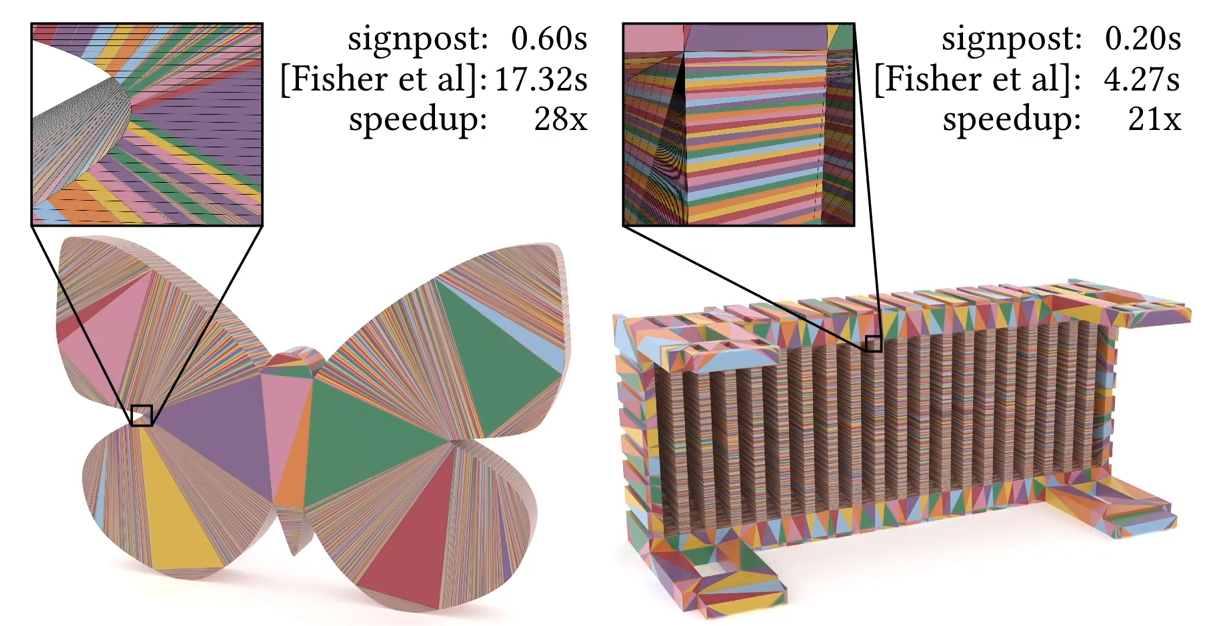also our implicit structure is much easier to work with which allows us to implement more advanced operations

and algorithms which weren't possible with previous data structures the most important of which is intrinsic Delaunay refinement.

although the intrinsic triangulation you get from edge flipping has some very useful properties it could still have very skinny triangles or a poor sampling of vertices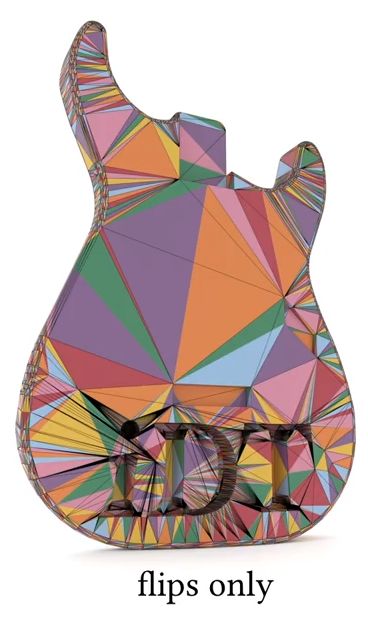Delaunay refinement addresses this by judiciously inserting new vertices into the triangulation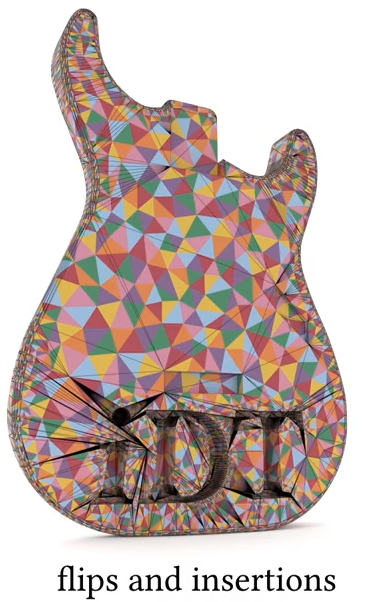the mesh on the right is not just Delaynay but also the smallest angle of the triangulation is 30 degrees and it has a reasonable sampling of vertices.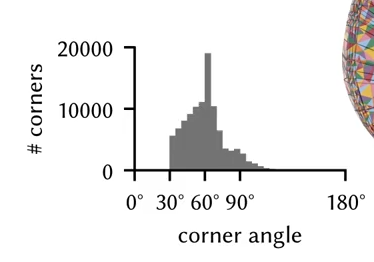once again we find that the algorithm from the plane works pretty much out of the box on surfaces. here we use an intrinsic version of Chew’s 2nd algorithm

repeatedly flipping edges until the mesh is Delauney while also inserting vertices at the circumcenter of skinny triangles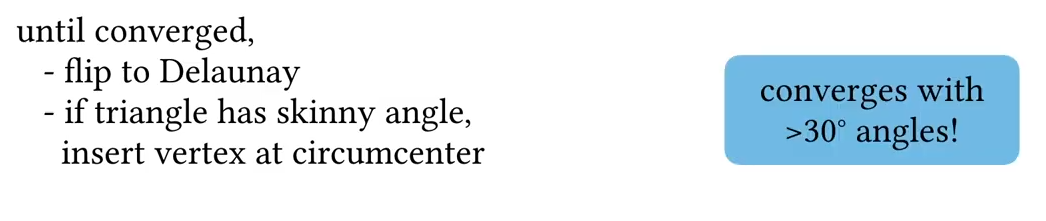In the plane this algorithm is known to converge to triangulations with angles no skinnier than 30 degrees or so and we observe essentially the same behavior on surfaces.

one tricky detail that shows up on surfaces is how to find the circumcenter of an intrinsic triangle.

in an acute triangulation this is easy  The circumcenter is just some point inside the triangle: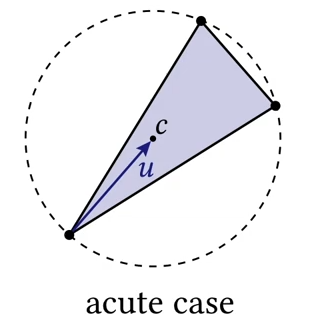but for an obtuse triangle the circumcenter might lie outside the triangle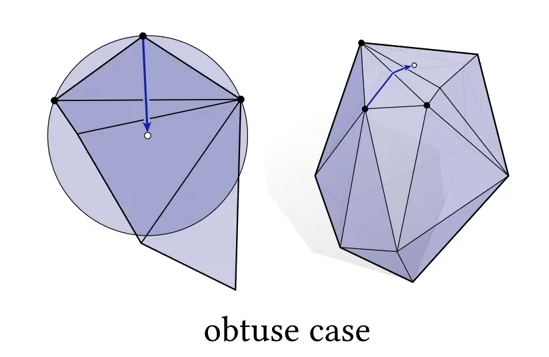here we construct a vector which points towards the circumcenter and trace out that vector along the surface wherever the tracing ends we insert a new vertex at that location

here are some examples of intrinsic Delaunay refinements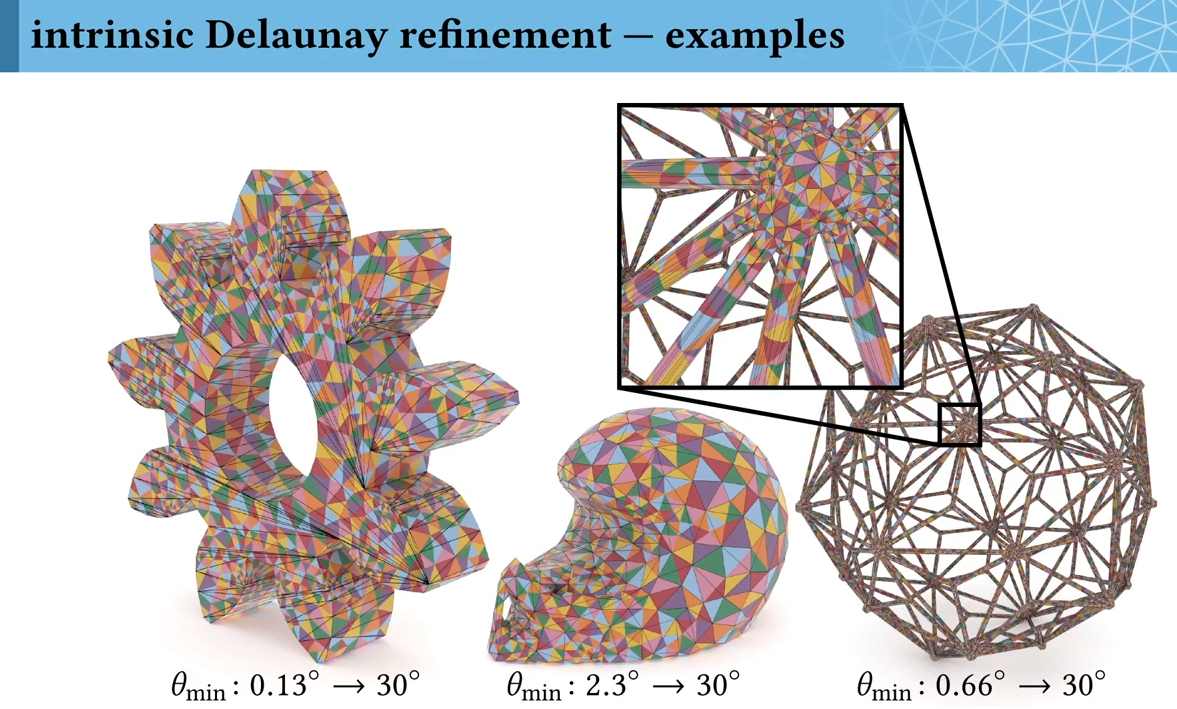these are really win-win meshes not too many elements good angle bounds the geometry is exactly preserved the algorithm runs in a fraction of a second

This is really exciting to me because for the first time triangulation of surfaces is starting to look like triangulation in 2d. There are no tolerances no trade-offs no relaxation you just ask for a nice mesh and you immediately get one

we have to slightly generalize how we think about the problem in order to get there but we really got something awesome in return.

You can actually use these intrinsic triangulations to implement all kinds of other triangulation algorithms beyond what I've already described

in the paper we further explored optimal Delaunay triangulations and also generating Steiner trees by inserting new vertices to induce shorter minimum spanning trees see the paper for more details

Now let me convince you that these intrinsic triangulations are actually easy to make use of in practical algorithms. The intrinsic triangulation you're working with might sit atop the input geometry in complicated ways, however, the implicit representation we expose is always simple and easy to use. It's just a mesh which has edge lengths and areas and all of this data you're used to using in your algorithms. the general pipeline will always be:

build a good intrinsic triangulation

run some existing algorithm on it

and then copy the result back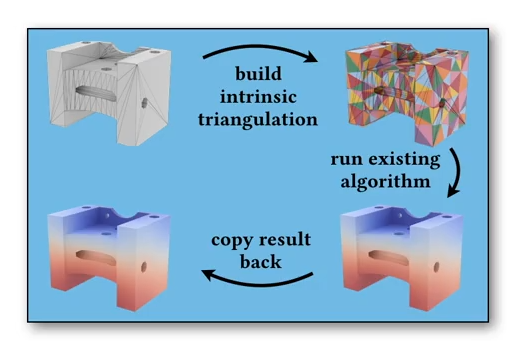In the examples I'm about to show that's all we did!

we built an intrinsic triangulation using one of the schemes I described previously (like intrinsic Delaunay refinement)

we naively ran some existing algorithm on that intrinsic triangulation

and then we copied the result back

as a warm-up suppose you need to solve a Poisson problem on a mesh

you could run our intrinsic version of two second algorithm to get a good intrinsic Delauney refinement

then the Laplace operator for the intrinsic triangulation and solve the linear system there

This turns out to be twice as accurate as the best extrinsic remeshing scheme we could come up with for the same budget of elements.

For many applications you can do even better by doing more work on the intrinsic triangulation. as a more interesting PDE based problem. consider using the heat method to compute geodesic distance which basically amounts to solving two PDEs running this procedure directly on

This badly triangulated input mesh results in more or less total garbage with 60% error from a ground truth solution. just performing intrinsic edge flips and running the same algorithm already reduces error significantly down to about 20% but running our intrinsic Delauney refinement works wonders taking error down to just 0.7 percent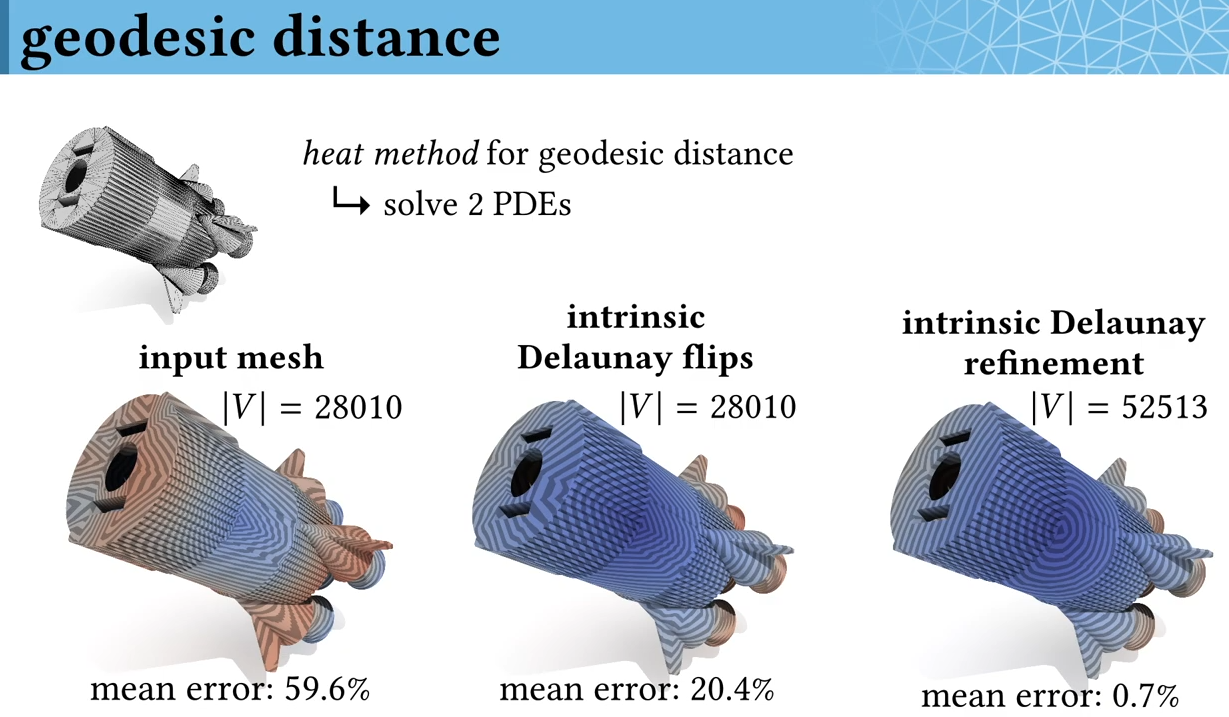this process is simple fast and didn't even need to double the number of vertices

-------------------------------

Our data structure also makes it easy to process tangent vectors. here we smoothly interpolate tangent vector data defined on the boundary into the interior of the shape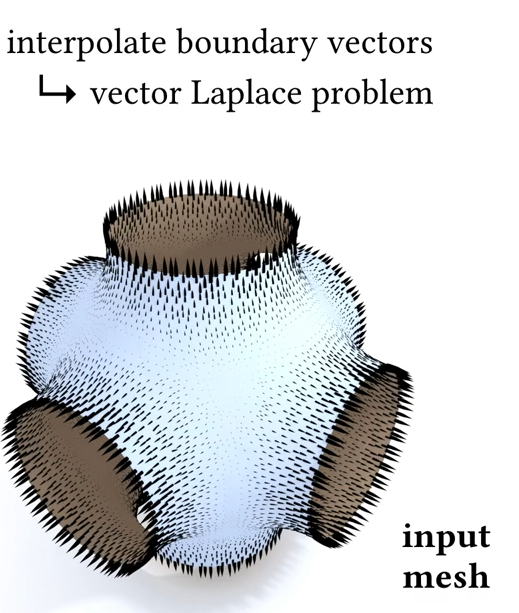doing this naively can result in flip vectors which point in the opposite direction you would expect which I've highlighted here in red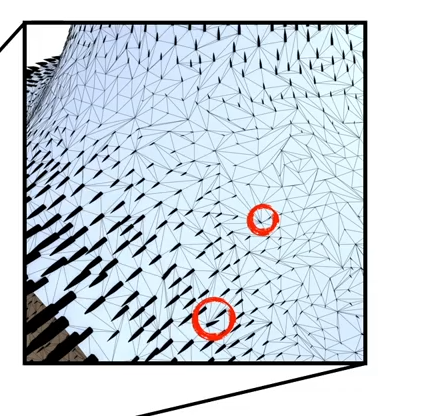this happens due to negative weights of the Laplace operator for the original mesh running this exact same procedure on our intrinsic triangulation will never ever yield these flip vectors and we can prove that via a maximum principle for the vector Laplace operator

again here's another vector field problem where we're simply looking for the smoothness vector field on a surface: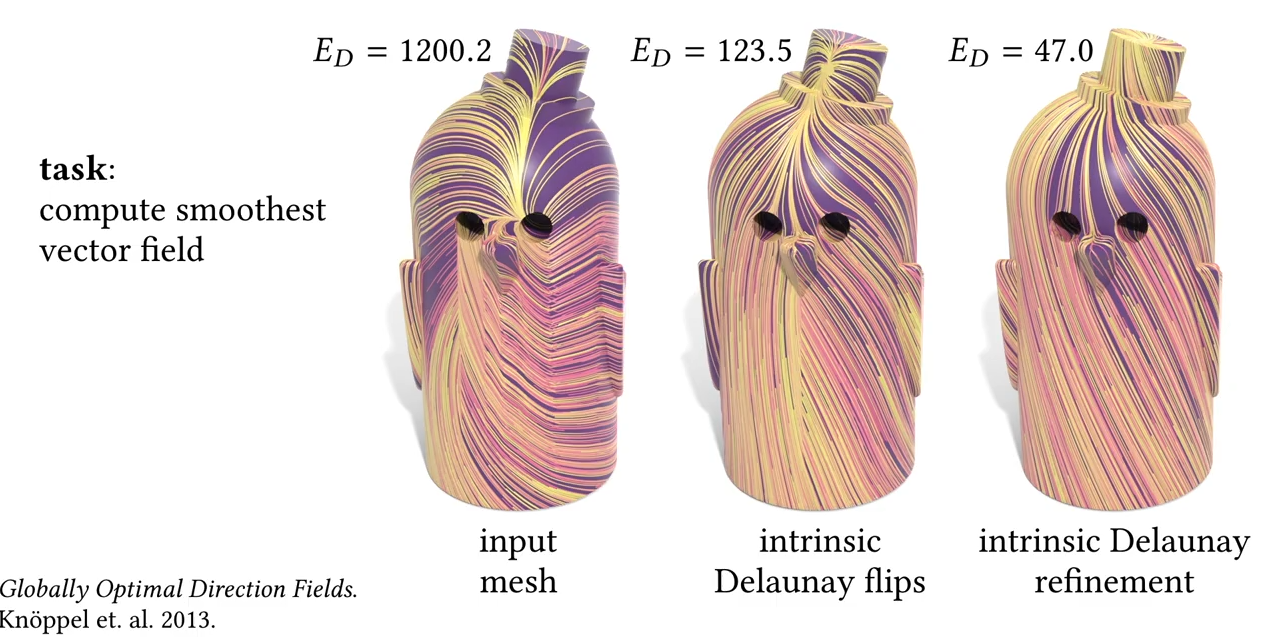running the exact same field generation algorithm on a good intrinsic triangulation yields a vector field which is visibly much smoother and quantitatively has much lower Dirichlet energy

We can push this even farther and do adaptive mesh refinement of surfaces. This is a technique widely used in scientific computing to great effect, however, it hasn't seen much use on curved surfaces

The idea is to refine the mesh only where a solution is interesting. (Adaptive Mesh Refinement AMR)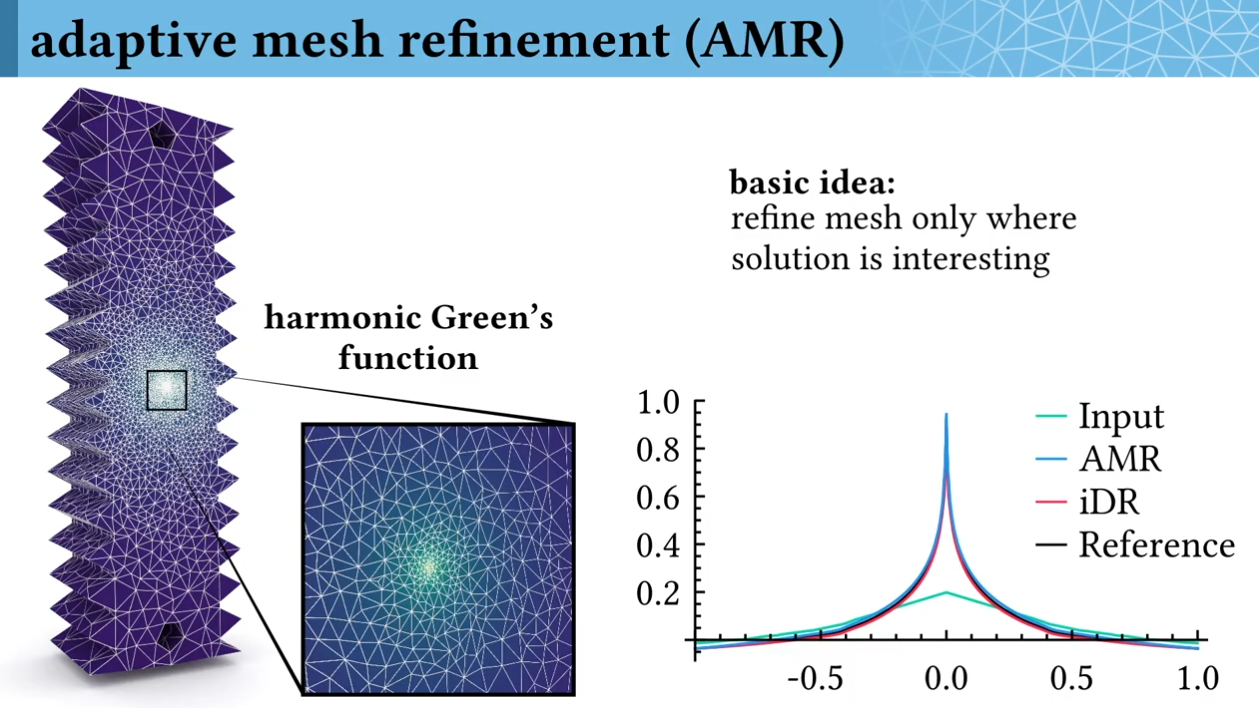Here this process nicely resolves a harmonic Green's function about a point.

We can use Amr to resolve parameterization problems too. here naively using a harmonic embedding yields a non injective resultsimply performing Delaunay edge flips ensures the parameterization is injective any everywhere by giving the laplacian a maximum principle :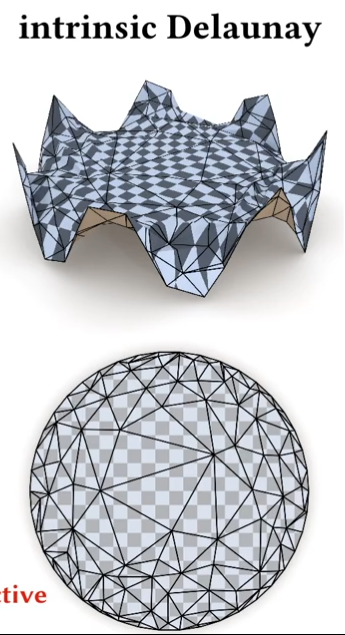and further running Amr with refinement resolves the solution well everywhere :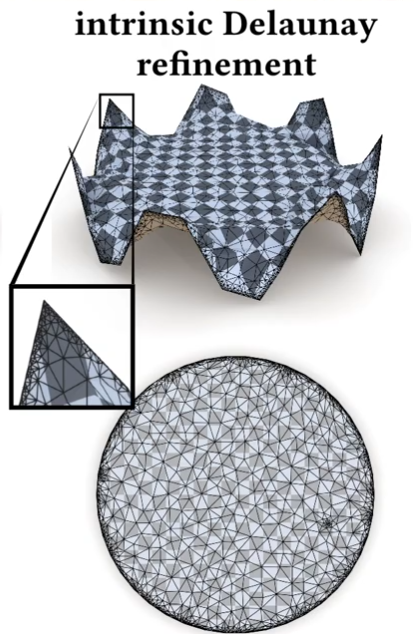looking forward there's a lot still to do with intrinsic triangulations:

Computational geometry of intrinsic triangulations

There's a big need for work on the computational geometry of these intrinsic triangulations. Do algorithms retain their same guarantees as in the plane?

Rational arithmetic, exact predicates

Similarly planar geometry has escaped even the pitfalls of floating point via the use of exact predicates and we'd love to do the same with surface triangulations to push robustness even further.

Intrinsic mesh simplification

One operation we didn't explore is intrinsic simplification, and that could probably be very useful in the context of surfaces.

Extension to non-manifold inputs

Since we're doing surface processing here we work solely with manifold meshes but it seems feasible to extend many of these techniques to the non manifold case.

I only scratched the surface here of potential uses for these intrinsic triangulations. This paradigm makes perfect sense for classes of algorithms like:

• spectral methods where all you really need to do is nicely resolve a spectrum of some operator
• for a surface learning where you want invariance to an input triangulation
• for compression where the ability to change the connectivity of a surface without changing its geometry is very useful

and so many more problems.

We've provided a nice code base which will allow you to check out some of these algorithms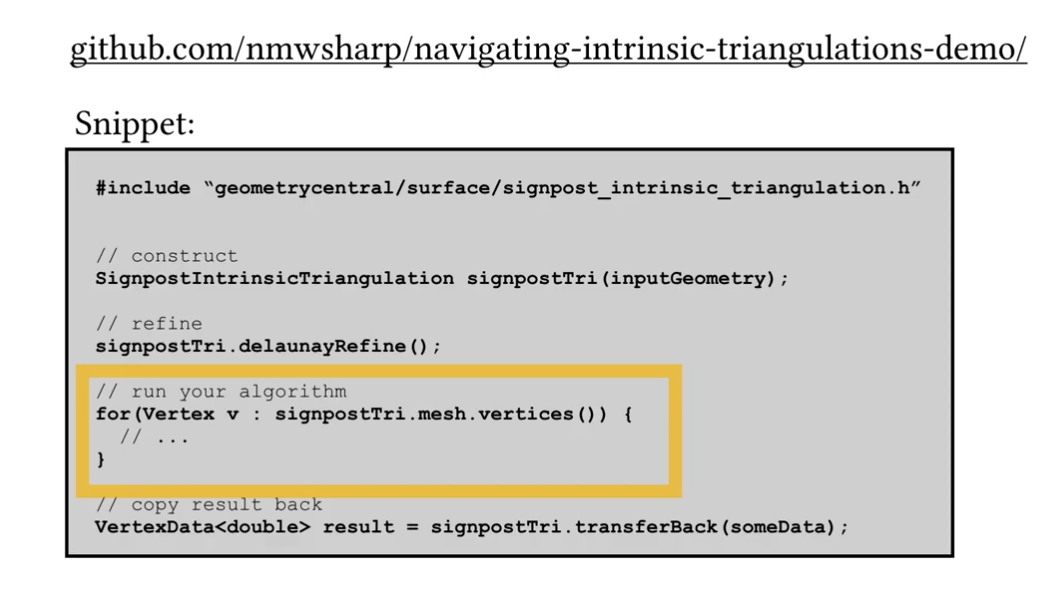https://github.com/nmwsharp/navigating-intrinsic-triangulations-demo

https://geometry-central.net/

and the important point here is that we exposed an intrinsic triangulation in a way that works just like a normal mesh. So it's really easy to map your algorithms onto this data structure!

As a closing thought, we're very optimistic about the future where robustness can simply be a subroutine in geometric algorithms. Rather than having to stress about numerical robustness for every single algorhythm you write, a huge class of these methods can instantly become robust in general; just by running on our intrinsic triangulations. So please please give us a try and let us know what you think. With that I'll close and thank you for your time.